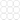## liangbch的地砖

Tue, 28th January 2020Edit on Github覆盖铺地砖

2008年7月liangbch提出一个铺地砖问题:

1.必须是采用正多边形的砖来铺，可以用一种正多边形，也可以采用几种正多边形。

2.每个顶点（多变形各个顶点的交叉点）上的多边形的排列必须相同。
3.采用相同的排列方式，地砖可无限扩展。反例，附件5-5-10 采用每个顶点包含 2个5边形和1个10边形，但是接着铺下去就不能满足条件了。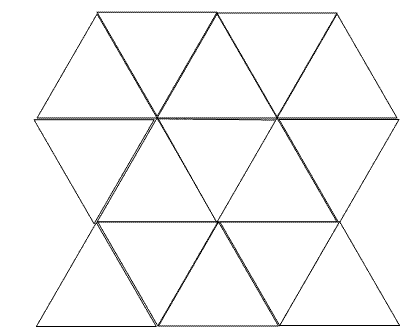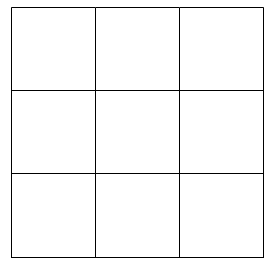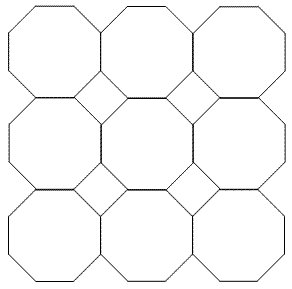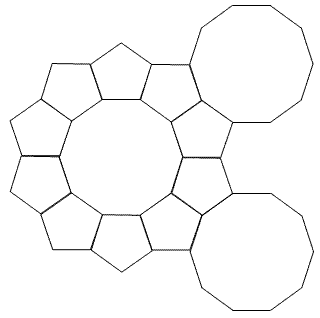# 更多讨论

liangbch提出

每个顶点3块砖
1、6+6+6
2、8+8+4(每个顶点包含2个八边形一个四边形,下同）
3、10+10+5

4、4+4+4+4
5、3+4+4+6

6、3+3+3+3+6

7、3+3+3+3+3+3

gxqcn指出，再增加两个：

　　8、4+6+12
9、3+12+12

$n$边形的一个内角对圆周角的“贡献”值为：$a_n = \frac{n-2}{2n}$

liangbch为gxqcn的方案配了对应的图 4+6+12: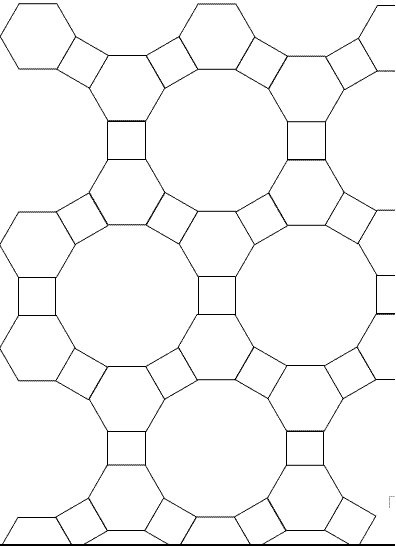3+12+12: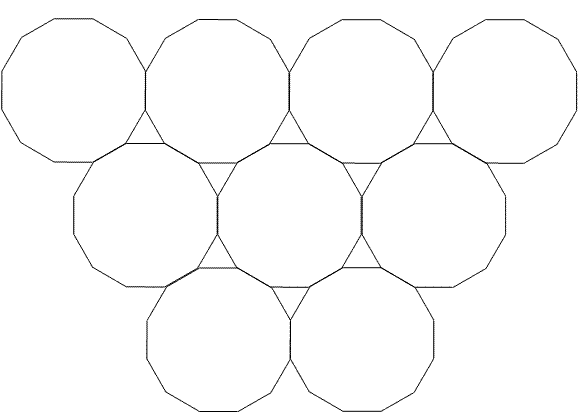三个角共点的有：
3+7+42
3+8+24
3+9+18
3+10+15
3+12+12
4+5+20
4+6+12
4+8+8
5+5+10
6+6+6

3+3+4+12
3+3+6+6
3+4+4+6
4+4+4+4

3+3+3+3+6
3+3+3+4+4

1、 如果同一个顶点的三个多边形中，有1个是奇数边，则另外两个多边形必须是 相同的偶数多边形。

　　符合条件的是：3+12+12
不符合条件被排除在外的是：
3+7+42
3+8+24
3+9+18
3+10+15
4+5+20
5+5+10

2、同一个顶点的三个多边形中全部是偶数边，且内角和为360°，则可以铺地板，如

　　　　4+6+12
4+8+8
6+6+6

3-3-3-3-6：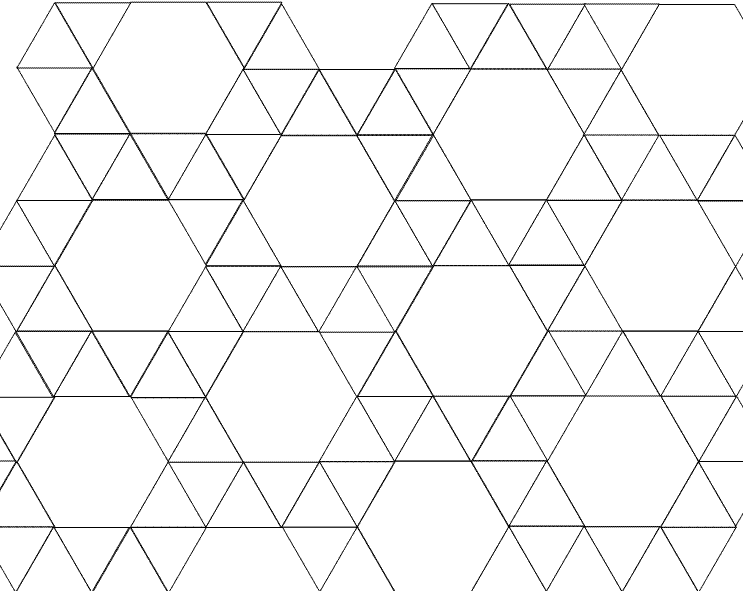3-4-6-4: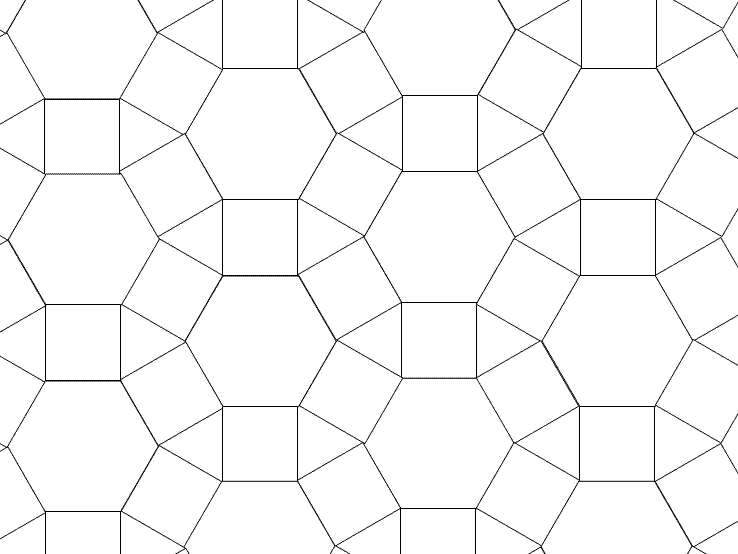3-6-3-6: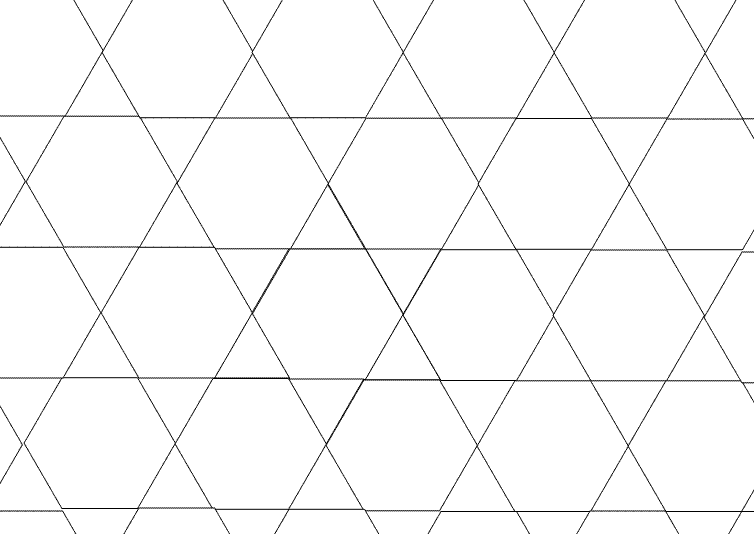3-3-6-6试图按（3，3，6，6）的顺序铺地板，结果导致了一些顶点（红圈标出）不再是(3,6,3,6)的顺序，是一种不符合条件的排法: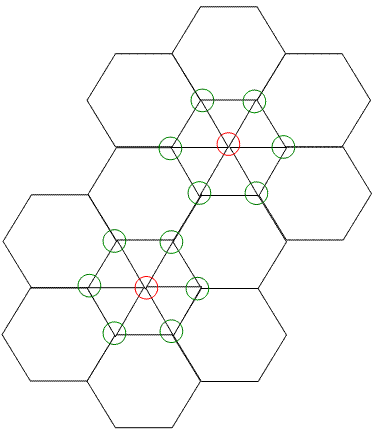3+12+12
4+6+12
4+8+8
6+6+6

3+6+3+6
3+4+6+4
4+4+4+4

3+3+3+3+6
3+3+3+4+4

3+3+3+3+3+6

shshsh_0510认为非周期的会更加漂亮，给出了44333型非周期方案: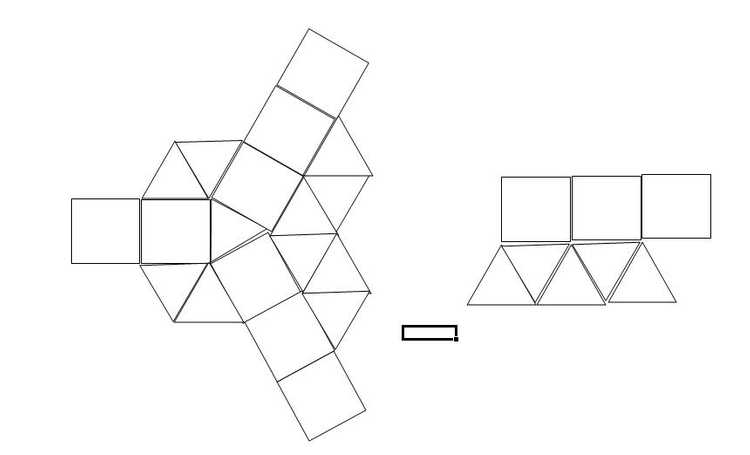Github